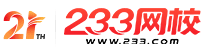#计算机二级

•扫描二维码进入

• 题 库扫描二维码进入

• 移动233网校移动

• 移动APP扫描二维码下载APP

## 2019年3月计算机二级MySQL基础试题及答案(6)

1.有以下程序：

#include

int abe(int u，int v);

main()

{ int a=24，b一16，c;

c=abc(a，b);

printf("%d\n"，c);

}

int abc(int u，int v)

{ int w;

while(v)

{w=u%v;u=v;v=w;)

return;

}

A.6

B.7

C.8

D.9

2.下列程序的输出结果是( )。

int fl(int x，int y){return x>y?x：y;)

int f2(int x，int y){return x>y?y：x;}

main()

{ int a=4，b=3，c=5，d=2，e，f，g;

e=f2(f1(a，b)，f1(c，d));

f=f1(f2(a，b)，f2(c，d));

g=a+b+c+d-e-f;

printf("%d，%d，%d\n"，e，f，g);}

A.4，3，7

B.3，4，7

C.5，2，7

D.2，5，7

3.语句int(*ptr)()的含义是( )。

A.ptr是一个返回值为int的函数

B.ptr是指向int型数据的指针变量

C.ptr是指向函数的指针，该函数返回一个int型数据

D.ptr是一个函数名，该函数的返回值是指向int型数据的指针

4.下述程序的输出结果是( )。

#include

main()

{ char chE2]一{”1234”，”5678”}，*p;

int i，j，s=0;

for(i=0;i<2;i++)

p[i]=ch[i];

for(i=0;i<2;i++)

{ s=p[i][j]一'0';

printf("%d"，s);}

}

A.6357

B.6135

C.1357

D.691357

5.下述程序的输出结果是( )。

#include

main()

{ int a={{1，2，3)，{4，5，6}}，(*p)，i;

p=a ;

for(i=0;i<3;i++)

{ if(i<2)

p[i]=p[i]一1;

else

p[i]=1;)

printf("%d\n"，a+a+a);}

A.8

B.7

C.12

D.9

6.若有以下说明，则( )不是对strcpy库函数正确的调用。

strcpy库函数用于复制一个字符串：char*strl="abcd"，str2，*str3="hijklmn"，*str4，*str5="aaaa";

A.strcpy(str2，strl)

B.strcpy(str3，strl)

C.strcpy(strl，str2)

D.strcpy(str5，strl)

7.执行下述程序后，输出的结果是( )。

#include

#define S(X)X*X

void main()

{ int a=9，k=3，m=2;

a/=S(k+m)/s(k+m);

printf("%d"，a);

}

A.1

B.4

C.9

D.0

8.已知有如下结构体：

struct sk

{ int a;

float b;

}data，*p;

A.(*p).data.a

B.(*p).a;

C.p->data.a

D.p.data.a

9.下述程序的输出结果是( )。

#include

void main()

{ int b={2，4，6，8，10，12);

int*p=b，**q=&p;

printf("%d，"，*(p++));

printf("%d，"，**q);

}

A.4，4

B.2，2

C.4，5

D.2，4

10.以下程序的输出结果是( )。

main()

{ int i，k，a，p;

k=5;

for(i=0：i<10;i++)a[i]=i;

for(i=0;i<3;i++)p[i]=a[i*(i+1)];

for(i=0;i<3;i++)k+=p[i]*2;

printf("%d\n"，k、;

}

A.20

B.21

C.22

D.23

• 海量免费模拟试题，在线测试。

• 各科每年真题试卷，实战演练！

• 逐章逐节针对练习，专项突破。

• 每天10道最新试题，随机练习。

• QQ群号：806931213

扫描二维码加群• ##### 微信订阅号：kszx233

扫描二维码加群